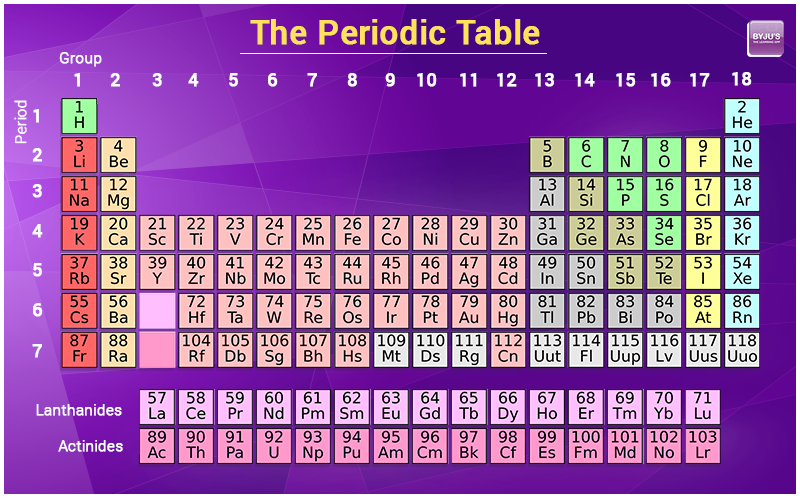# Atomic Mass Formula

Atomic mass in an atom or group of an atom is the sum of the masses of protons, neutrons and electrons. The electrons have very less mass in comparison to protons or neutrons so the mass of electrons is not influenced in the calculation. For an element, relative atomic mass is the average mass of the naturally occurring isotopes of that element relative to the mass of an atom of 12C. Which means one atom is given a relative atomic mass of exactly 12. Atomic mass is not reported with units. The formula for atomic mass is given below

Atomic mass = Mass of protons + Mass of neutrons + Mass of electrons

## How to Calculate Atomic Mass?

There are three ways to calculate the atomic mass, depending on the circumstances of each.

### 1. By referring to the periodic table

In the periodic table digit of an atomic mass usually marked under the representation of an element. For example

Hydrogen (H) atomic mass -1

Lithium (Li) atomic mass -3Periodic Table

### 2. Addition Of Mass Of Protons and Neutrons

In an element atomic mass of an atom can be calculated by adding the mass of protons and neutrons.

### 3. All Atoms of an Element – Weighted Average

3. Weight average of an element

The weighted average of the isotopes of each element grounded in nature by their abundance is the atomic weight of an element. One can calculate the atomic mass of the element with these tips. A list of isotopes with natural abundance and mass is given either as a percentage or decimal value. Each isotopes-abundance is multiplied by its mass. If isotope abundance is present their solution should be divided by 100 and add these values.
The solution means the atomic mass of the specific element. the atomic mass unit can be related with the other mass unit by using the conversion factor.1u = 1.66054 X 10-24g.

## Atomic Mass of Elements

The atomic mass of a few substances is tabulated below.

 Substance Atomic mass Calcium ion 40.1 Chloride Ion 35.5 Magnesium Ion 24.3 Potassium Ion 39.1

## Relative Atomic Mass

The link between the mass of an element and the number of atoms it contains is the relative atomic mass of the element. By using this chemists work out the chemical formula. The relative atomic mass scale is used to compare the masses of different atoms.

First the hydrogen atom the lightest atom was originally assigned a relative atomic mass of 1 and the relative atomic mass of other atoms was compared with this.

 Element Relative atomic mass Carbon 12 Hydrogen 1 Oxygen 16 Magnesium 24 Nitrogen 14

## Atomic Mass Problems

Problem – 1Find the element mass number whose atomic number is 18 and the neutron number is 20.

Solution:

Number of protons = 18
Number of Neutrons = 20
Atomic mass Number = Number of protons + number of neutrons
A = 20 + 18
Mass number (A) = 38
 More topics in Atomic Mass Formula Avogadro’s Law Formula Molecular Weight Formula Weighted Average Formula Gay Lussac Law Formula